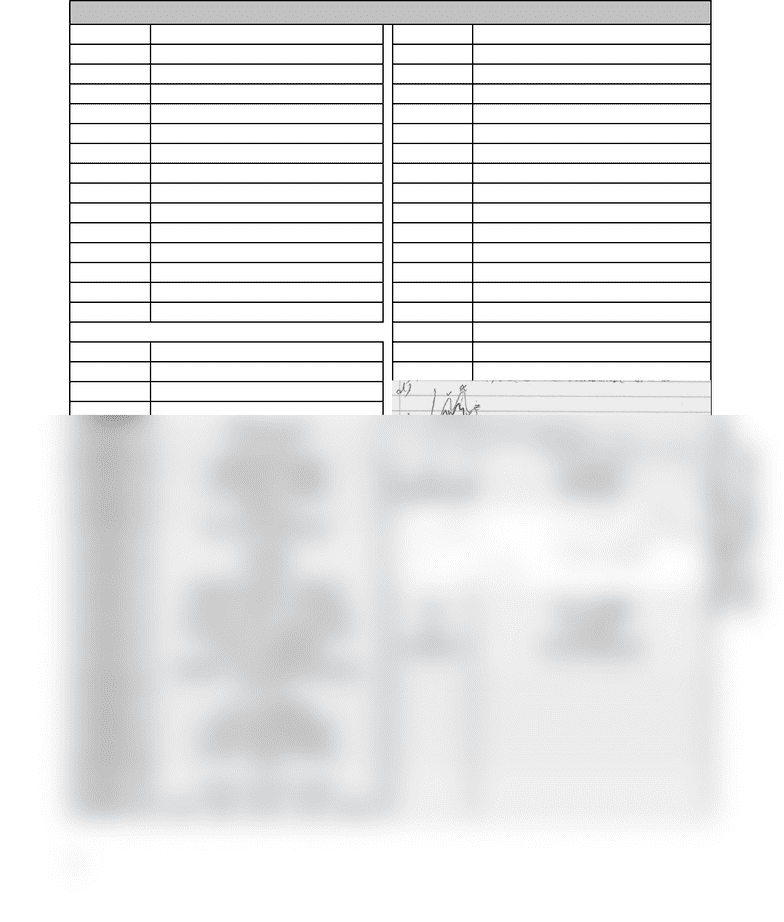Study Guides (390,000)
CA (150,000)
UTSG (10,000)
CHM (100)
all (30)
Midterm

# CHM139H1 Midterm: CHM139 TT2 Package 1 Soln (2015 F)

Department
Chemistry
Course Code
CHM135H1
Professor
all
Study Guide
Midterm

This preview shows half of the first page. to view the full 2 pages of the document.(1.1) Ex. 1 C (4.1 x 10
-5
M/s) (1.6) Ex. 1 A (1.2 x 10
-12
)
(1.1) Ex. 2 B (-2/3 d[O
2
]/dt] (1.6) Ex. 2 D (182 kJ/mol)
(1.1) Ex. 3 2.4 x 10
-4
M/s(1.6) Ex. 3 D (Incr. fraction to overcome E
a
)
(1.1) Ex. 4 B (CH
4
rate is 1/3 H
2
rate)(1.6) Ex. 4 C (176 kJ/mol)
(1.1) Ex. 5 0.46 M/s (1.6) Ex. 5 B (-19
o
C)
(1.2) Ex. 1 C (M
-1
s
-1
)(1.6) Ex. 6 E (More energy > E
a
)
(1.2) Ex. 2 A (2nd order) (1.6) Ex. 7a) rate = k[I
2
][H
2
]
(1.2) Ex. 3 D (Factor of 3) b) I
(1.2) Ex. 4 D (1/M s
2
)c) 52.9 kJ/mol
(1.2) Ex. 5 C (rate = k[A][B]) (1.6) Ex. 8a) rate = k[C
2
F
4
]
2
(1.2) Ex. 6 A (2.8 x 10
-2
M
-1
s
-1
)b) k = 0.0448 M
-1
s
-1
(1.2) Ex. 7 C (rate = k[A]
2
[B]
1
)c) [C
2
F
4
]
o
= 0.543 M
(1.2) Ex. 8 D (rate = k[A]) d) [C
4
F
8
]
= 0.0665 M
(1.2) Ex. 9a) K = 1.8 x 10
-3
M
-1
s
-1
e) 54.52 times faster
b) Yes - bimolecular process. (1.7) Ex. 1a) 3 steps
No, difficult rearranging system of molecules.
b) W --> Y
c) Ea = 186kJ/mol c) V, X, Z
d) [HI] = 0.2545 M d) and e) See below
(1.3) Ex. 1 C (t
1/2
indep. of [A])
(1.3) Ex. 2 A (0.02572 M)
(1.4) Ex. 1 E (100 hours)
(1.4) Ex. 2 5.12 x 10
-7
s
(1.4) Ex. 3 A (0th order, 0 balls) f) Increases k
1
(1.4) Ex. 3 A (0th order, 0 balls) f) Increases k
(1.4) Ex. 4 C (14 black, 2 white) (1.7) Ex. 2a) See below
(1.4) Ex. 5 C (621 s)
(1.4) Ex. 6 B (14.7% consumed)
(1.4) Ex. 7 E (18 s)
(1.4) Ex. 8 A (0.94)
(1.5) Ex. 1 E (Orders indep. of stoich.)
(1.5) Ex. 2 D (Tetra. molec. rxn unlikely) b) rate = k[A][B]
(1.5) Ex. 3 C (Orders det. by experiments) c) No effect
(1.5) Ex. 4 E (rate = k[H
2
][NO]
2
)(1.7) Ex. 3 B (Increasing [A])
(1.5) Ex. 5 D (Mech. 2 only with bimolecular)
(1.5) Ex. 6 A (I, IV)
(1.5) Ex. 7 A (I
-
= Catalyst)
(1.5) Ex. 8 A (rate will not change)
(1.5) Ex. 9 D (rate = k[Cl]
1/2
[CHCl
3
])
(1.5) Ex. 10 B
(1.5) Ex. 11 C (Int. = Cl, Cat. = N/A)
(1.5) Ex. 12 C (2H
+
+ 2Br
-
+ H
2
O
2
—> Br
2
+ 2H
2
O)
1
Copyright 2015 Toronto Life Sciences (TLS)
www.TLifeSci.com